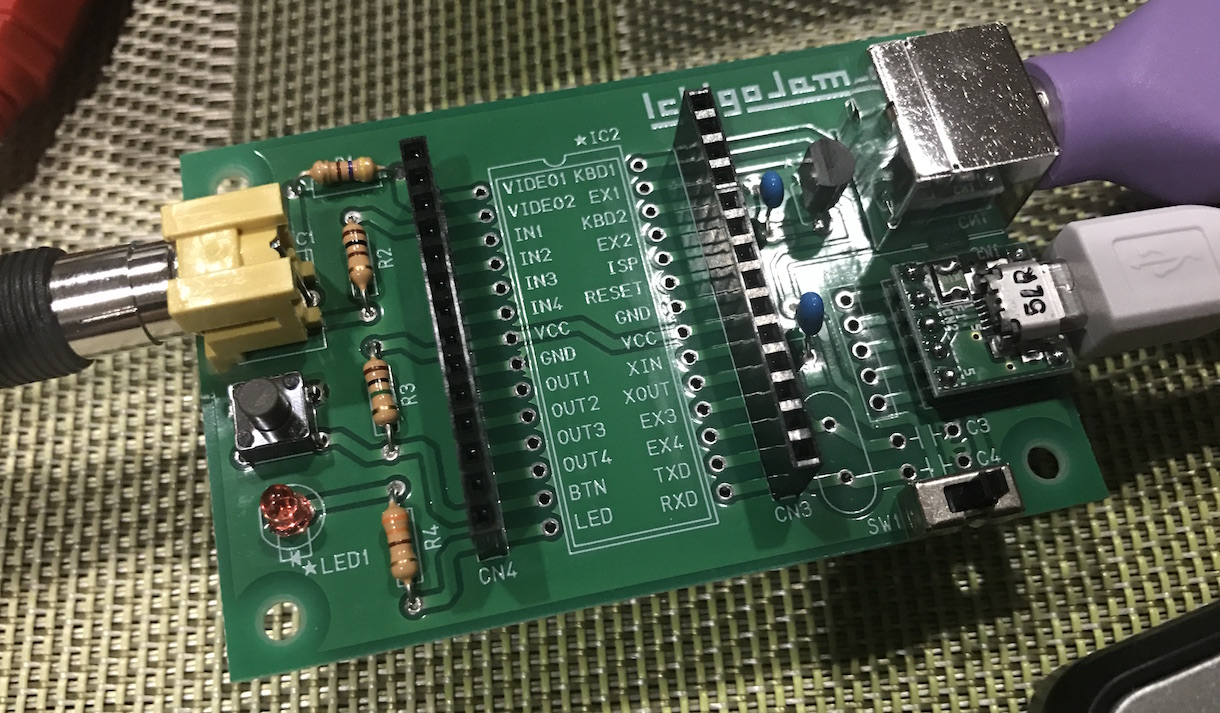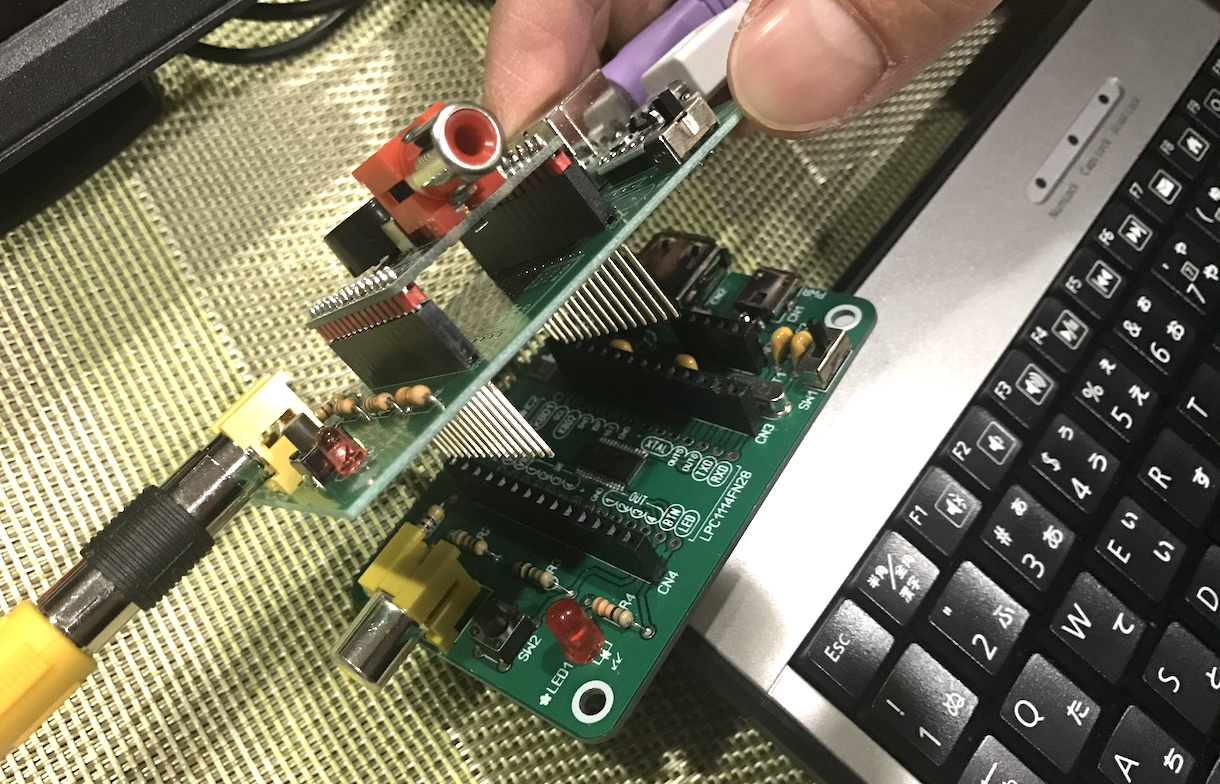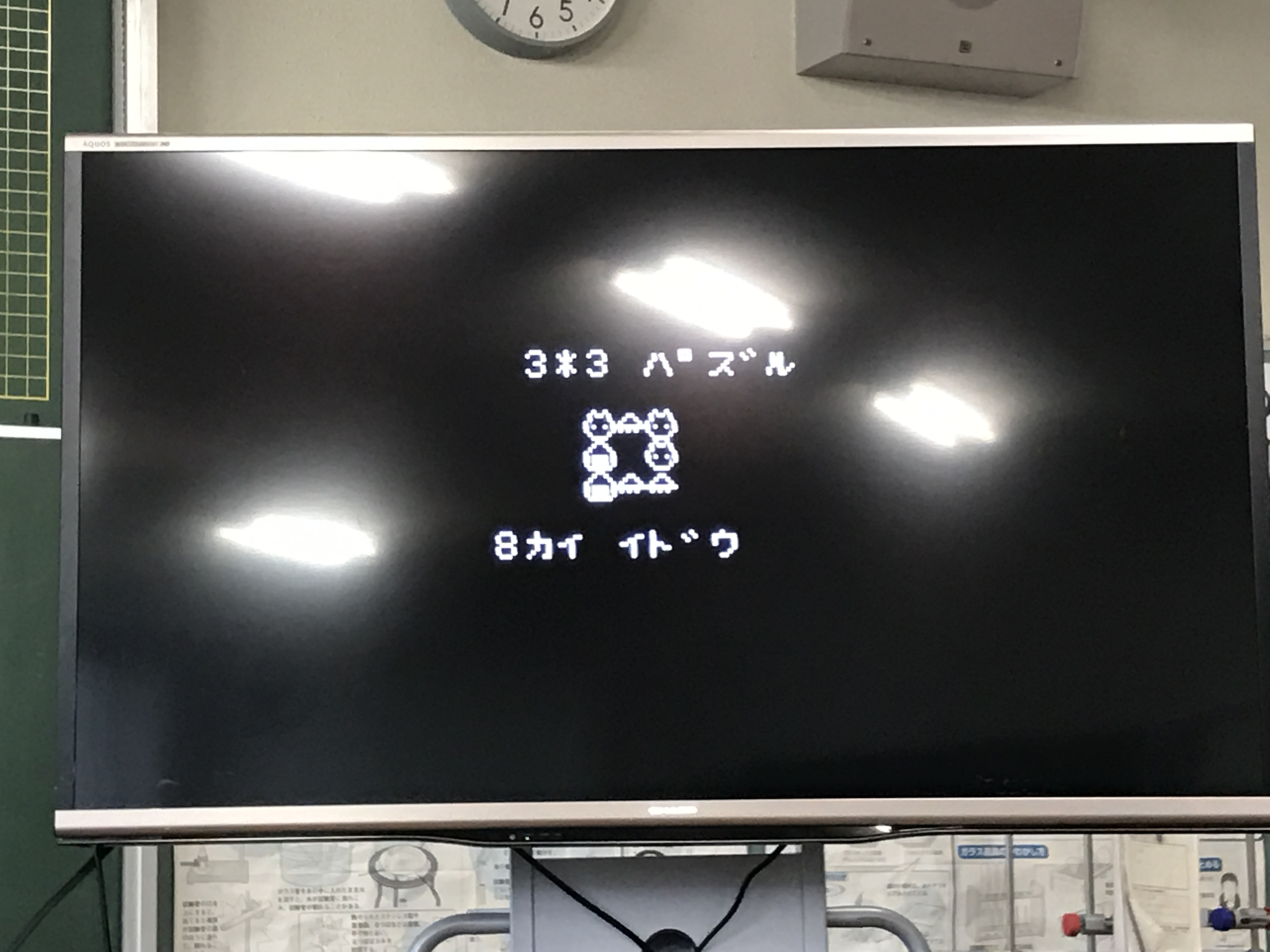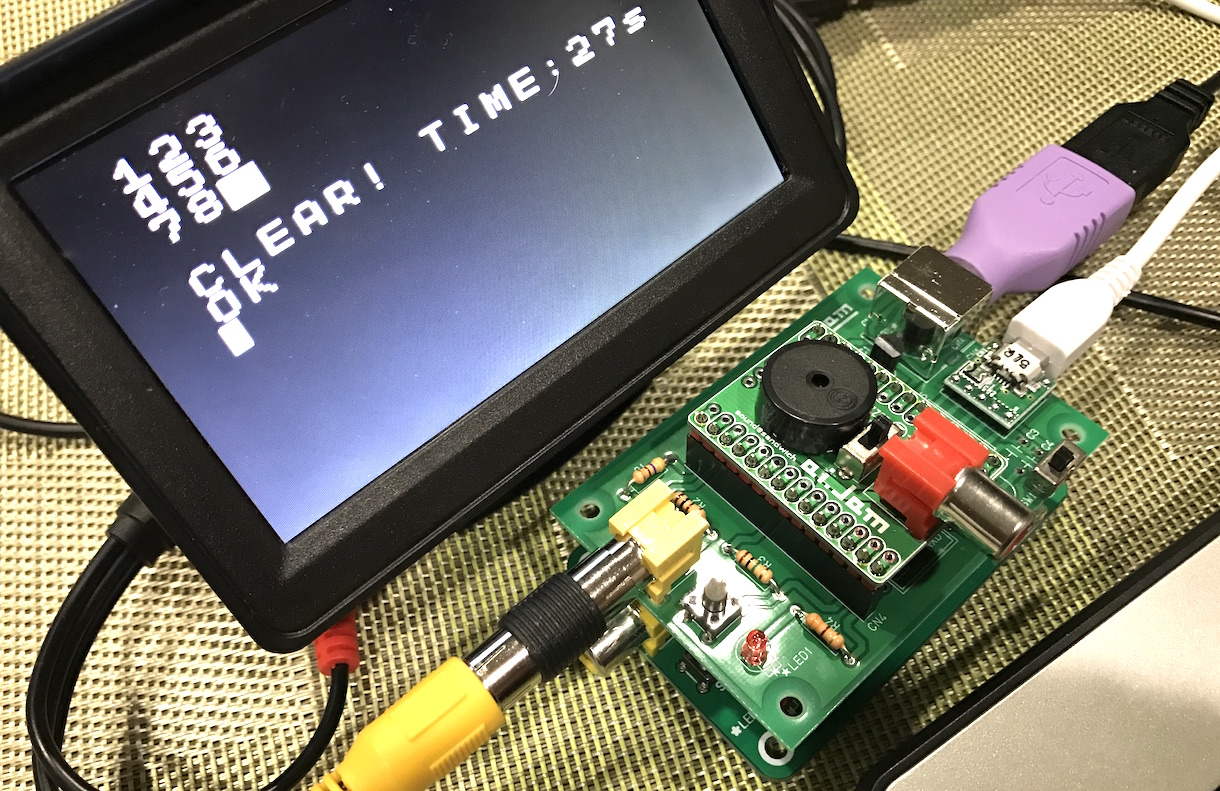2017-01-31
I showed presentation about programs of the club of Sabae Junior Highschool (鯖江中学校).
It's very fun! Thank you!!

One of presentations. I understood students like to combine games!I made a presentaiton shield for IchigoJam for quick preparations.It was made by IchigoJam parts without CPU and long pin socket.I was asked a question to make puzzle.

So I made a sample 3x3 puzzle.10 CLS:FOR I=0 TO 7:LC I%3,I/3:?I+1:NEXT 20 X=2:Y=2:FOR I=1 TO 200:K=RND(4)+28:GOSUB 60:NEXT:CLT 30 LC X,Y:?"*" 40 F=0:FOR I=0 TO 7:F=F+(SCR(I%3,I/3)=I+49):NEXT:IF F=8 LC0,4:?"CLEAR! TIME:";TICK()/60;"s":PLAY"O5E16E":END 50 K=INKEY():GOSUB 60:GOTO 30 60 LC X,Y 70 X=X-(K=28)*(X>0)+(K=29)*(X<2):Y=Y-(K=30)*(Y>0)+(K=31)*(Y<2) 80 ?CHR\$(SCR(X,Y)):RETURN

Let's try!CC BY / @taisukef / アイコン画像 / プロフィール画像 / 「一日一創」画像 / RSS